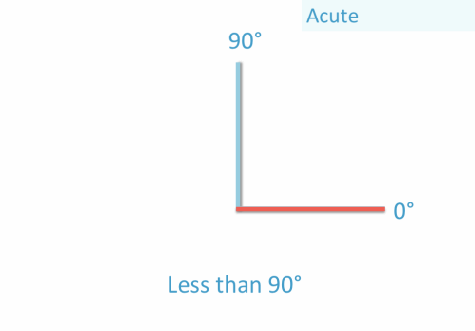# Faces, Edges and Vertices of 3D Shapes

Faces, Edges and Vertices of 3D Shapes• 3D means three dimensional.
• Three dimensional shapes can be picked up and held because they have length, width and depth.
• Faces are the surfaces on the outside of a shape.
• Edges are the lines where two faces meet.
• Vertices (or corners) are where two or more edges meet.

3 Dimensional shapes have length, width and depth.

The properties of a 3D shape are the number of faces, edges and vertices that it has.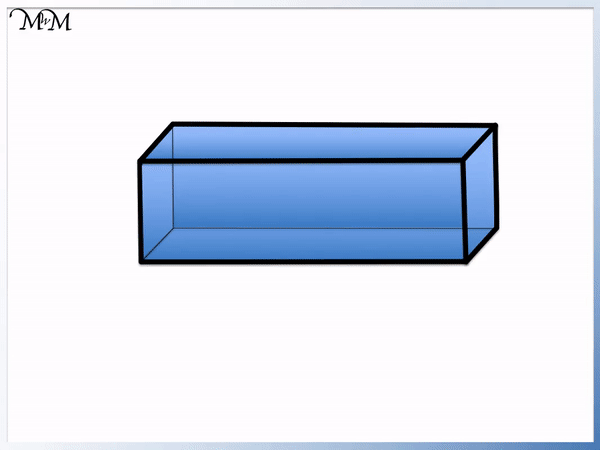• The above 3D shape is a cuboid, which is box shaped object.
• A cuboid has 6 rectangular faces, which are the outside surfaces of a 3D shape.
• A cuboid has 12 straight edges, which are the lines between the faces.
• A cuboid has 8 vertices, which are its corners where the edges meet.
• A cuboid has exactly the same number of faces, edges and vertices as a cube.
• A cuboid is different from a cube in that its edges are longer in at least one direction, whereas a cube has edges that are all equal in length.Supporting LessonsAccompanying Lesson Activity Sheet: Nets of 3D Shapes

# Faces, Edges and Vertices of 3D Shapes

## What are the Properties of 3D Shapes?

The properties of 3D shapes are faces, edges and vertices.

• Faces are the flat or curved surfaces that make up the outside of a 3D shape.
• Edges are the lines where two faces on a 3D shape meet.
• Vertices are the corners of a 3D shape formed where two or more edges meet.

For example, a cube has 6 faces, 12 edges and 8 vertices.

The poster below shows the faces, edges and vertices of 3D shapes labelled on a cube.The following table lists the number of faces, edges and vertices for some common 3D shapes:

Name Faces Edges Vertices
Cube 6 12 8
Cuboid 6 12 8
Sphere 1 0 0
Cylinder 3 2 0
Cone 2 1 1
Square-Based Pyramid 5 8 5
Triangular-Based Pyramid 4 6 4
Sphere 1 0 0

3D is short for three-dimensional. All three dimensional shapes have the the three dimensions of length, width and depth.

A shape is 3D if it can be picked up and held in real-life.

When teaching the properties of 3D shapes, it is worth having a physical item to look at as you identify and count each property.

There are also printable nets for each 3D shape above that can be downloaded and assembled to accompany this lesson. Alternatively, there are some online interactive 3D shapes in the practice section above that you can use to count the faces, edges and vertices.

When teaching this topic, it can be helpful to count the number of each property on the net before assembling it.

You can colour in each face a different colour, or write a number from 1 – 6 on each square face. You can mark each edge as you count it by drawing a line on each one. You could put a sticker or piece of plasticine on each vertex as you count it.

Marking the faces, edges and vertices as you count them is important as it can be easy to count them twice or miss one out.

## Faces, Edges and Vertices of a Cube

A cube has 6 faces, 12 edges and 8 vertices. Each face of a cube is a square. All of its edges are the same length.Each of the 6 faces of a cube is square-shaped because all of its edges are the same size. A cube is a 3D square.

There are 12 edges on a cube, which are all the same length. There are 4 horizontal edges around both of the top and bottom square faces. There are also 4 vertical edges connecting the top square face to the bottom square face.There are 8 vertices on a cube. There are 4 vertices on the top square face and 4 vertices on the bottom square face.

## Faces, Edges and Vertices of a Cuboid

A cuboid has 6 faces, 12 edges and 8 vertices. Each face of a cuboid is a rectangle. It is an elongated cube.

A cuboid is a 3D box shape and it has rectangular faces. A cuboid is also known as a rectangular prism.A cuboid has 6 rectangular faces. The opposite faces on a cuboid are equal in size.

A cuboid has 12 edges. It has 4 horizontal edges around the top rectangular face and 4 horizontal edges around the bottom rectangular face. It also has 4 vertical edges connecting the vertices of the top rectangular face to the 4 vertices of the bottom rectangular face.

A cuboid has 8 vertices. It has 4 around the top rectangular face and 4 around the bottom rectangular face.A cuboid has the same number of faces, edges and vertices as a cube. This is because a cube is a special type of cuboid that has all of its edges the same size.

The difference between a cube and a cuboid is that a cube has equal edge lengths, whereas a cuboid is longer in at least one direction.

When teaching 3D shape names, it is worth comparing a cube and cuboid alongside each other to identify the differences between the two.

The opposite faces on a cuboid are equal and can be coloured in the same colour on your net.

## Faces, Edges and Vertices of a Sphere

A sphere has 1 curved surface, 0 flat faces, 0 edges and 0 vertices. A sphere is a 3D circle.

A sphere is ball-shaped and is perfectly round, which means that it is not longer in a particular direction than any other.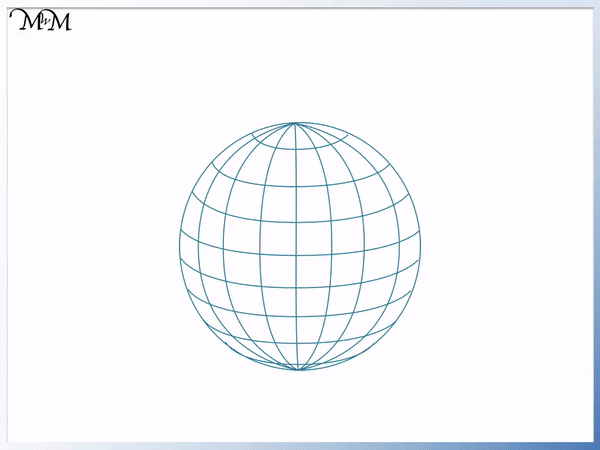A sphere contains no flat faces but it has one continuous curved surface. A sphere is a shape that contains no edges or vertices. This means that it feels smooth to touch all the way around.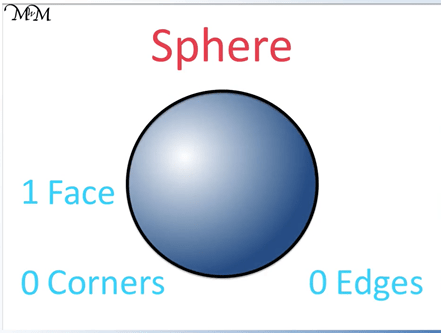It can help to pick up a spherical object and feel for edges and vertices. Whilst the net may be useful to help visualise the shape, we recommend using a ball or perfect sphere for this exercise as the net will be very difficult to make spherical with no clear edges or vertices.

## Faces, Edges and Vertices of a Cylinder

A cylinder has 2 flat faces, 1 curved surface, 2 circular edges and no vertices. The top and bottom faces are flat and circular. A curved surface wraps around the outside of these 2 faces.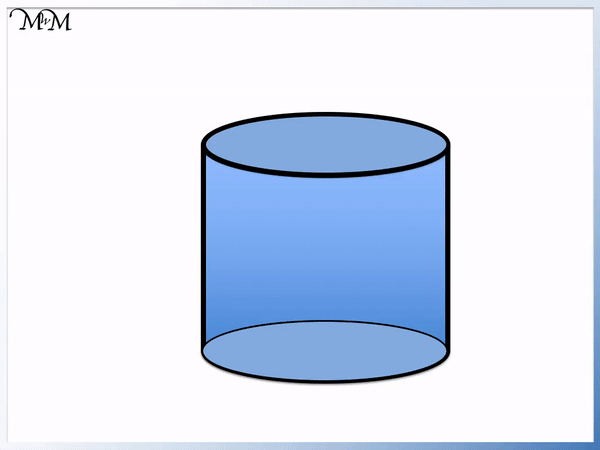The cylinder has two identical circular faces on the top and bottom. When viewed from the top or bottom, a cylinder looks circular.

A cylinder has 2 curved edges that wrap around the circular faces on the top and bottom of the shape.

A cylinder has no vertices. This is because it has only 2 edges and the 2 edges do not meet each other.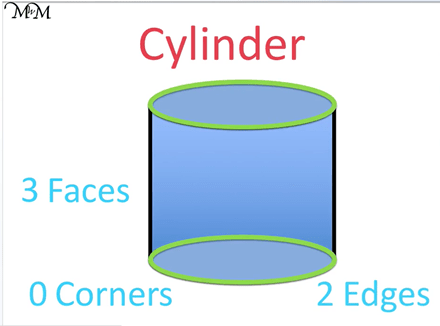The curved face of a cylinder forms a rectangle when it is rolled out flat and the net of a cylinder can help to show this.

Everyday objects that are cylinders include food tins and batteries. Toilet rolls are also common cylindrical objects but they do not have the top and bottom circular faces that a true cylinder should have.

With cylinders containing circles and spheres being rounded in appearance, it can be common to mix these two shape names up. The easiest way to tell the difference between a cylinder and a sphere is that a cylinder has edges.

It is worth comparing the two alongside each other and feeling the edges on a cylinder, which the sphere does not have.

Both a cylinder and a sphere will roll since they both contain a curved face. Spheres will roll in every direction, however, the cylinder will only roll in one direction when placed on its side.

## Faces, Edges and Vertices of a Square-Based Pyramid

A square-based pyramid contains 5 faces, 8 edges and 5 vertices. The bottom face is a square and there are also 4 more triangular faces around the side of the shape. There are 4 vertices around the square base plus one more on the tip of the pyramid.A square-based pyramid contains 5 faces. The base is a square face and there are 4 triangular faces around the sides. These 4 triangular faces meet together at the tip of the pyramid.

The square-based pyramid contains 8 edges. There are 4 horizontal edges around the square base and 4 more sloping edges between each triangular face.

The square-based pyramid contains 5 vertices. There are 4 around the square base and one more at the tip of the pyramid.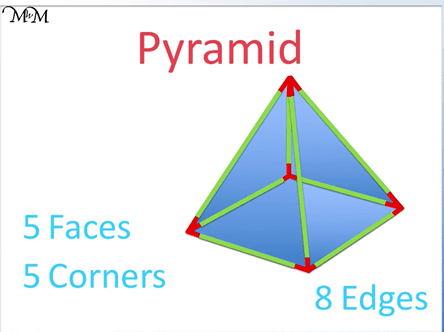The Egyptian pyramids are examples of real-life square-based pyramids.

There are several types of pyramid, which are named by the face of the base.

## Faces, Edges and Vertices of a Cone

A cone contains 1 flat circular face, 1 curved surface, 1 circular edge and 1 vertex. The vertex is formed from the curved surface and it is directly above the centre of the circular base.A cone contains 1 flat circular face on its base. It also has a curved surface wrapping around this curved base. Technically it has 1 face in total but often the curved surface is included in the count to make 2 faces.

A cone contains 1 circular edge that wraps around the bottom circular face.

A cone contains 1 vertex which is on the very top of the shape directly above the centre of the circular base. It is formed from the curved surface.It is possible that your child may mix a cone up with either a cylinder or a pyramid.

The difference between a pyramid and a cone is that a cone has a circular base and can roll on its side.

A cone and a cylinder both contain a circular base and you can hold the completed nets up and look directly at their base faces to see that they look identical from this orientation.

The cone converges to a point, whereas the cylinder does not.

You can compare how they roll to see the difference between them. A cone rolls in a circle because one end is wider than the other. A cylinder rolls in a straight line.

Traffic cones and ice-cream cones are common examples of the cone shape in real-life.Now try our lesson on Classifying Angles as Acute, Obtuse, Right or Reflex where we learn how to describe angles.